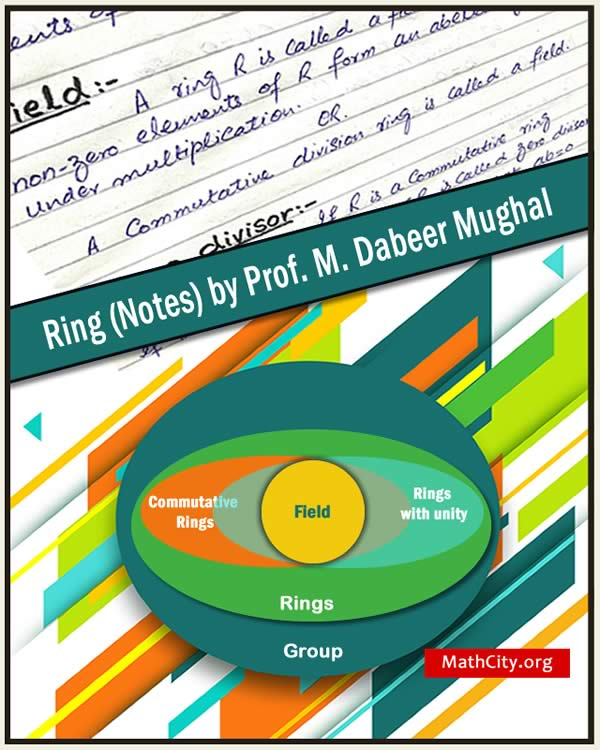# Ring (Notes) by Prof. M. Dabeer MughalA handwritten notes on Ring (Algebra) by Prof. M. Dabeer Mughal (Federal Directorate of Education, Islamabad, Pakistan). It is best to prepare a “Rings and Vector Spaces” section of your algebra paper or Algebra II for BS or MS Mathematics.

 Name Ring (Notes) - Handwritten Prof. M. Dabeer Mughal 64 pages PDF & DJVU (see Software section for viewers) PDF: 4.90MB DJVU: 3.8MB
• Rings, commutative ring, ring with unity (identity), examples
• Consequences from the definition
• Unit element of ring
• Division ring or skew field, field, zero divisor
• Integral domain
• Characteristic of ring, Boolean ring
• Sub-ring and related theorem
• Centre of ring and related theorem
• Ring homomorphism
• Isomorphism, kernel of $\phi$ and related theorems
• Ideal, left ideal
• Right ideal, two sided ideal, examples and theorems
• Principal ideal, principal ideal ring and related theorem
• Quotient ring and related theorem
• Ist fundamental theorem
• Maximal ideal and related theorem
• Prime ideal and related theorem
• The field of quotients of an integral domain
• Prime field

• notes/ring_notes